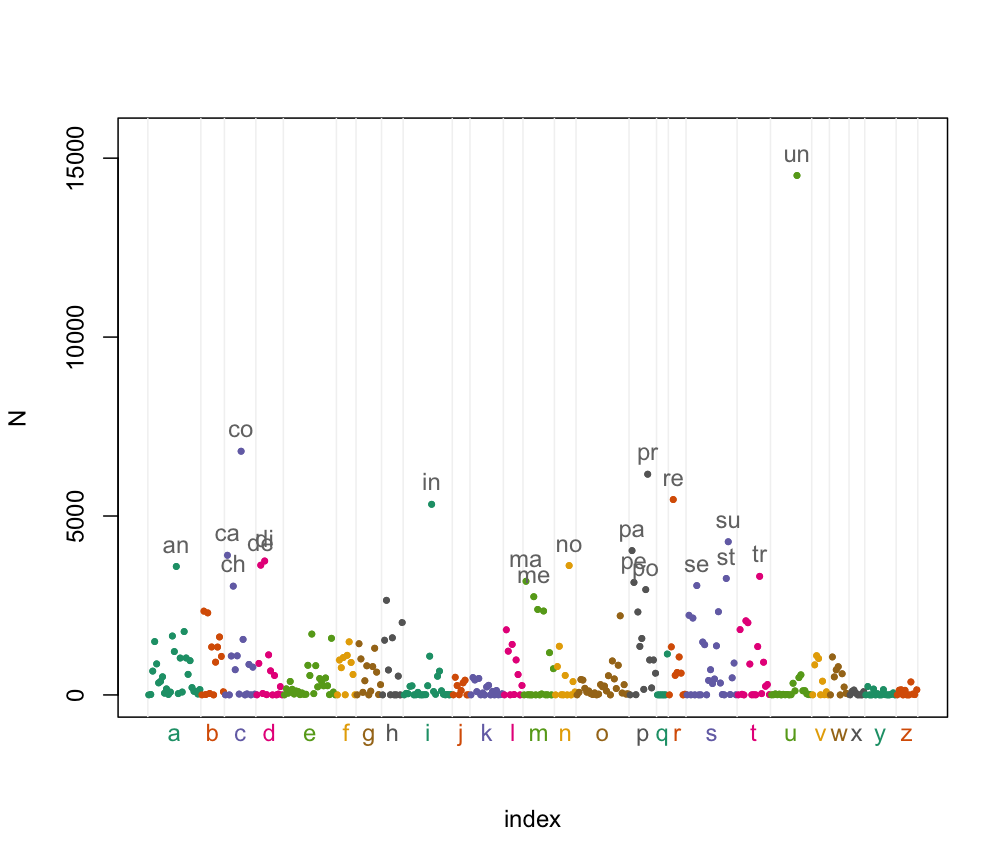# A Manhattan plot of the words of English

A Manhattan plot refers to the graphical display of GWAS results across the chromosomes. Here is a Manhattan plot of the first two letters of the words of English.

```## Print a dictionary as a Manhattan plot. Steve Bagley, Sept 2015.

library(data.table)
library(RColorBrewer)

setnames(words, "V1", "word")
## only use letters
words[, word := gsub("-", "", word, fixed = TRUE)]
## store bigram only using lowercase
words[, bigram := tolower(substr(word, 1, 2))]
## count bigram occurrences
tab <- words[, .N, by = bigram]
## build percentiles
dist_fn <- tab[, ecdf(N)]
tab[, percentile := dist_fn(N)]
tab[, initial := substr(bigram, 1, 1)]
## get colors. palette only has 8 colors, so wrap around to cover alphabet
plot_colors <- rep(brewer.pal(8, "Dark2"), length.out = 26)
## color is set by initial letter of bigram
tab[, color := plot_colors[strtoi(initial, base = 36) - 9]]
tab[, index := as.numeric(rownames(tab))]
## build plot, don't label x-axis yet, and make room for label of highest point
tab[, plot(index, N, col = color, pch = 19, cex = 0.5, xaxt = "n", ylim = c(0, 15500))]
## put the initial letter at the mean location of that letter
alphabet <- tab[, .(mean_loc = mean(index), min_loc = min(index), max_loc = max(index)), by = initial]
## some very light gray lines between the letters
alphabet[, abline(v = c(min_loc - 0.5, max(max_loc) + 0.5), col = "grey95")]
## draw them on x-axis, in color
alphabet[, mtext(initial, side = 1, at = mean_loc, col = plot_colors)]
## put the points back on top of background
tab[, points(index, N, col = color, pch = 19, cex = 0.5, xaxt = "n")]
## mark outliers with bigram label
tab[percentile > 0.95, text(index, N, labels = bigram, pos = 3, col = "grey45")]
```Created: 2016-04-30 Sat 15:43